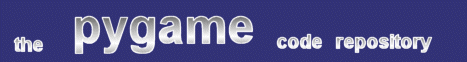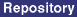### Title: Starfield Class

Author: David Clark (da_clark at shaw.ca)
Submission date: December 2, 2001

Description: starfield.py is a pretty solid class for rendering a parallax starfield effect onto an arbitrary surface. You can set the number of stars, their angle, speed, color and size. You can render onto the entire surface, or just a certain portion of that surface. You can change the starfield's direction and speed on the fly.

Use the arrow keys. Up/Down changes the speed of the starfield, and Right/Left changes the direction in ten degree increments. Hit ESC to quit.

pygame version required: Any
SDL version required: Any
Python version required: 2.0

Comments: There are two flaws I can see in the code. First, the Star._calculate_axis_speeds() function is slow (although it's as fast as possible without forcing the user to specify angles in radians :)) The result is a visible pause when changing starfield direction or speed. The function was written to allow arbitrary angles; most games don't need that kind of precision. Try precalculating the values that you'll need before the game begins, then plugging them into this function during gameplay, rather than recalculating these trig values every time the user hits a key.

The second flaw is that the stars are drawn in the order they're created, not in z-order from furthest to closest. Try setting the STAR_SIZE_IN_PIXELS to 10 to see the effect - more distant stars occasionally move over closer ones. This effect may be visible with any star size over 1, although at lower values, the effect will be negligible. Star sizes over 2 aren't recommended anyway, since the stars are square, not round :)

```
#! /usr/bin/env python

import whrandom, pygame, math

class Star:
""" Star is a crippled particle class - it can draw itself, keep track
of its speed, position and direction, color and size. """

def __init__(self, position_x, position_y, distance, angle, speed, size,
color):
self.position_x = position_x
self.position_y = position_y
self.distance = distance
self.size = size
self.angle = angle
self.speed = speed
self._calculate_axis_speeds()
self.color = (int(distance * color),
int(distance * color),
int(distance * color))

def draw(self, display):
display.fill(self.color, (self.position_x, self.position_y,
self.size, self.size))
return

def erase(self, display):
display.fill((0,0,0), (self.position_x, self.position_y,
self.size, self.size))
return

def move(self, elapsed_time):
self.position_x += (self.speed_x * elapsed_time)
self.position_y += (self.speed_y * elapsed_time)
return

def set_angle(self, new_angle):
self.angle = new_angle
self._calculate_axis_speeds()

def set_speed(self, new_speed):
self.speed = new_speed
self._calculate_axis_speeds()

def _calculate_axis_speeds(self):
# This is a pretty slow function - performance is poor because of
# the math. If you had a game where the starfield could only move
# in certain directions, or at certain speeds, you might be able to
# increase performance by precalculating the values you need, and
# feeding them in as constants.
self.speed_x = math.cos(self.angle / (180 / math.pi)) * self.speed
self.speed_y = math.sin(self.angle / (180 / math.pi)) * self.speed

class Starfield:
"""
Starfield(display, rect, number_of_stars, angle, speed_sequence,
size, color)

The starfield class uses the following arguments:

display            -- A pygame surface
rect               -- A pygame.Rect on that surface - the starfield
might only take up a certain area on your
display, leaving room for a hud, etc.
number_of_stars    -- An integer - the more stars, the slower the
animation.
angle              -- From 0 to 360 in degrees
speed_sequence     -- A sequence of two integers. The first is the
speed of the slowest (most distant) star, the
second is the speed of the fastest (closest)
star. Both values are in pixels per second.
size               -- The size of the stars in pixels. One is the
default.
color              -- An RGB tuple of your stars' color. The
color of individual stars will vary from this
for the closest, to nearly black for the most
distant. This is optional - if it's omitted,
the closest stars will be bright white.

The starfield can have its angle and speed changed on the fly.
"""

def __init__(self, display, rect, number_of_stars, angle,
speed_sequence, size = 1, color = (255, 255, 255)):
self.display_surface = display
self.display_rect = rect
self.angle = angle
self.fastest_star_speed = speed_sequence
self.slowest_star_speed = speed_sequence
self.brightest_color = color
self.number_of_stars = number_of_stars
self.timer = Timer()

# create our stars

self.stars = []
for index in range(number_of_stars):
x_pos = self._random_x()
y_pos = self._random_y()
distance = whrandom.random()
speed = ((distance * \
(self.fastest_star_speed - self.slowest_star_speed)) + \
self.slowest_star_speed)

my_star = Star(x_pos, y_pos, distance, angle, speed, size, color)
self.stars.append(my_star)

def update(self):
self.erase()
self.move()
self.draw()

def draw(self):
for my_star in self.stars:
my_star.draw(self.display_surface)
return

def erase(self):
for my_star in self.stars:
my_star.erase(self.display_surface)
return

def move(self):
elapsed_time = self.timer.get_elapsed_time()
for my_star in self.stars:
my_star.move(elapsed_time)
# Check if the star has moved off a screen edge. If so, put it
# back on the opposite screen edge at a random position.
if my_star.position_x <= self.display_rect.left:
my_star.position_x = self.display_rect.right
my_star.position_y = self._random_y()
elif my_star.position_x >= self.display_rect.right:
my_star.position_x = self.display_rect.left
my_star.position_y = self._random_y()
if my_star.position_y <= self.display_rect.top:
my_star.position_y = self.display_rect.bottom
my_star.position_x = self._random_x()
elif my_star.position_y >= self.display_rect.bottom:
my_star.position_y = self.display_rect.top
my_star.position_x = self._random_x()
return

def set_angle(self, new_angle):
self.angle = new_angle
for star in self.stars:
star.set_angle(new_angle)
return

def set_speeds(self, new_speed_sequence):
self.fastest_star_speed = new_speed_sequence
self.slowest_star_speed = new_speed_sequence
for star in self.stars:
new_speed = ((star.distance * \
(self.fastest_star_speed-self.slowest_star_speed)) + \
self.slowest_star_speed)
star.set_speed(new_speed)
return

def _random_x(self):
return float(whrandom.randint(self.display_rect.left,
self.display_rect.right))

def _random_y(self):
return float(whrandom.randint(self.display_rect.top,
self.display_rect.bottom))

class Timer:
""" This class lets me scale a star's movement based on how much time
has passed since the previous frame.  That results in smoother, more
constant motion, even if the frame rate varies. """
def __init__(self):
self.current_time = pygame.time.get_ticks()

def get_elapsed_time(self):
now = pygame.time.get_ticks()
elapsed_time = (now - self.current_time) * 0.001
self.current_time = now
return elapsed_time

if __name__ == "__main__":
pygame.init()

# Change these for different effects

NUMBER_OF_STARS = 250
STARTING_ANGLE = 180
CLOSEST_STAR_COLOR = (255, 255, 255)
STAR_SIZE_IN_PIXELS = 1

# A few preset speeds for the user to see.

preset_speeds = ( (10, 1), (20, 2), ( 40, 4), (60, 6), (80, 8) )
current_speed = 2

# For fullscreen, replace pygame.SWSURFACE with pygame.FULLSCREEN

display = pygame.display.set_mode((640, 480), pygame.SWSURFACE)

my_starfield = Starfield(display, display.get_rect(), NUMBER_OF_STARS,
STARTING_ANGLE, preset_speeds[current_speed],
STAR_SIZE_IN_PIXELS, CLOSEST_STAR_COLOR)

while 1:
my_starfield.update()
pygame.display.update()

# Check for quit, and process user input (arrow keys)

for event in pygame.event.get():
if event.type is pygame.QUIT:
raise SystemExit
elif event.type is pygame.KEYDOWN:
if event.key is pygame.K_ESCAPE:
raise SystemExit
elif event.key == pygame.K_RIGHT:
new_angle = my_starfield.angle + 10
if new_angle > 360:
new_angle -= 360
my_starfield.set_angle(new_angle)
elif event.key == pygame.K_LEFT:
new_angle = my_starfield.angle - 10
if new_angle < 0:
new_angle += 360
my_starfield.set_angle(new_angle)
elif event.key == pygame.K_UP:
if current_speed != len(preset_speeds) - 1:
current_speed += 1
new_speed = preset_speeds[current_speed]
my_starfield.set_speeds(new_speed)
elif event.key == pygame.K_DOWN:
if current_speed != 0:
current_speed -= 1
new_speed = preset_speeds[current_speed]
my_starfield.set_speeds(new_speed)

```
 From: Anonymous Date: August 26, 2002 11:17 GMT A minor addition to the class is an updater that only updates the changed part of the surface. I was playing with this class and at 1280x1024, the updates become naturally sluggish. --- starfield.py.orig Sun Dec 2 19:09:13 2001 +++ starfield.py Mon Aug 26 07:08:33 2002 @@ -22,11 +22,15 @@ def draw(self, display): display.fill(self.color, (self.position_x, self.position_y, self.size, self.size)) + my_updater.addrect(self.position_x, self.position_y, + self.size, self.size); return def erase(self, display): display.fill((0,0,0), (self.position_x, self.position_y, self.size, self.size)) + my_updater.addrect(self.position_x, self.position_y, + self.size, self.size); return def move(self, elapsed_time): @@ -185,6 +189,23 @@ return elapsed_time +class Updater: + def __init__(self): + self.rects = [] + + def addrect(self,x,y,w,h): + self.rects = self.rects + [(x,y,w,h)] + return + + def clear(self): + self.rects = [] + return + + def get_rects(self): + return self.rects; + + + if __name__ == "__main__": pygame.init() @@ -207,10 +228,12 @@ my_starfield = Starfield(display, display.get_rect(), NUMBER_OF_STARS, STARTING_ANGLE, preset_speeds[current_speed], STAR_SIZE_IN_PIXELS, CLOSEST_STAR_COLOR) + my_updater = Updater(); while 1: + my_updater.clear(); my_starfield.update() - pygame.display.update() + pygame.display.update(my_updater.rects); # Check for quit, and process user input (arrow keys)

 Feedback